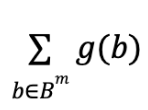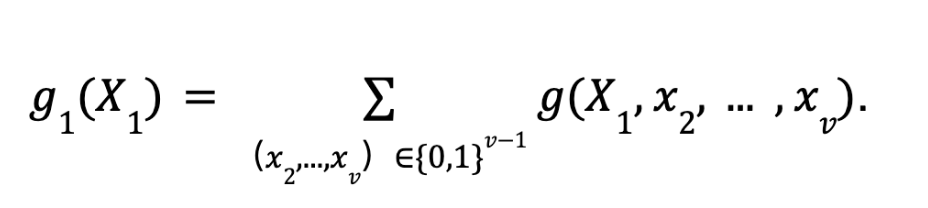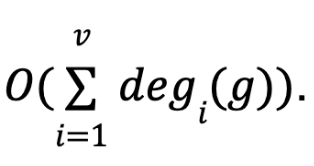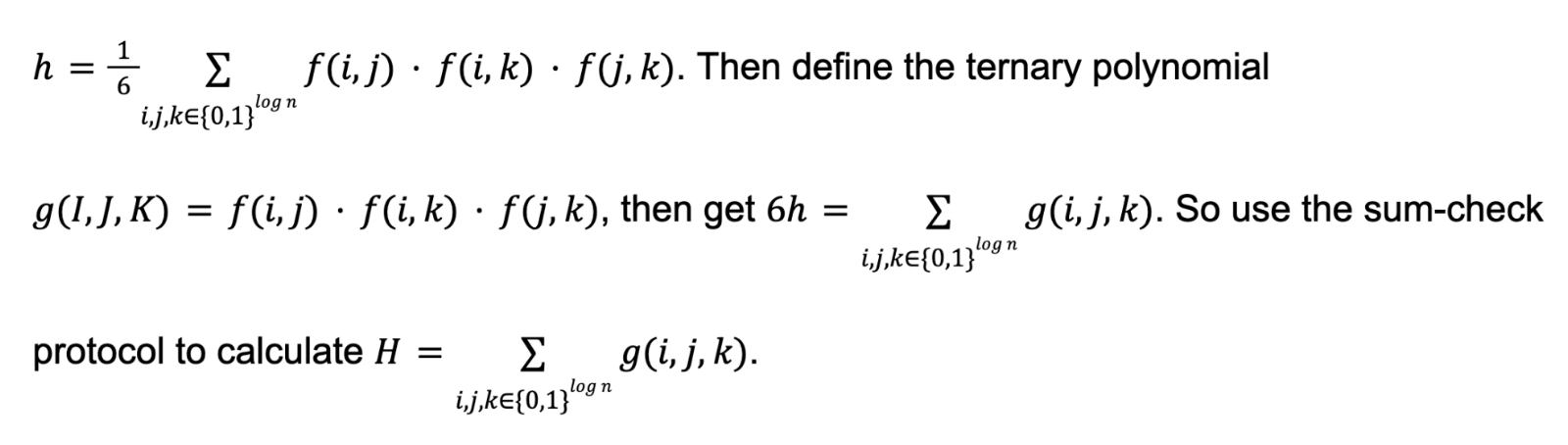# What is the Sum-Check Protocol of Zero Knowledge Proofby Fredericke KANG, CEO of Fox Tech, and Sputnik Meng, Chief scientist of Fox Tech

With the spread of Bitcoin, blockchain, smart contract and other concepts, more and more people pay attention to the vigorous development of Web3 field. On the technical side, many developers are also focusing on the cryptographic protocols underlying the blockchain. In this article, the zero-knowledge proof protocols play a unique role, both in the implementation of privacy protection and the implementation of the Layer2 zkRollup.

Zero-knowledge proof is a general term for a class of algorithms. So far, many protocols have been developed, including Plonk, Groth16, zkStark, Virgo, Orion, Foaks, and so on. Different protocols are applicable to different computing scenarios with varying complexity and efficiency. For example, Foaks has the advantage of linear proof time and small proof length.

In each of these protocols, the goal is the same: the Prover wants to convince the Verifier that it has a secret without revealing any information about it to the verifier. The sum-check protocol is a component of many protocols, which was first proposed in [LFKN92]. Many computation problems can be converted into problems that the sum-check protocol can handle, thus generating proofs. The underlying protocols of many protocols, including Foaks, are based on the sum-check protocol, on which adjustments are made.

It also plays an important role in the Foaks proof system used by Fox Tech. To be specific, in order to prove the correctness of an opcode, it is necessary to convert it into an arithmetic circuit first, then into a matrix, and finally generate a polynomial. The algorithm in the proof system is applied to the polynomial. In the final compression proof part, Also, the interaction between Prover and Verifier is transformed into a process that computes a sum, known as a sum-check protocol.

# Sum-check Protocol

## Protocol objective

The goal of the agreement is simple and easy to understand.

Let’s say we have a v-element polynomial defined over a finite field F, let’s call it g. The objective of the protocol is to calculate the sum:

Similar to the “outsourced computations” scenario considered in zkRollup, these computations can be very computable in the application, and we want to hand the computations to the Prover, who then validates the computations to the Verifier.

## Protocol assumption

First, we need to clarify the capabilities of the validator in this protocol. We assume that the verifier has an Oracle that computes the function g. That is, for the verifier to determine that an input  r1, … ,rv, to calculate g(r1, … ,rv) is easy. But calculating the complete result H is difficult.

In fact, in the real world’s application, Oracle does not exist, but it can be achieved in some way, for example, we can let the prover to help the verifier calculate the value, and add more tricks to the correctness of the proof.

Second, regarding the goal of the protocol, in fact, the sum-check protocol can calculate

##for any set b, but without loss of generality. Here we assume B={0,1}.

## Protocol procedure

The agreement consists of v rounds. One variable in g is processed in each round.

Round 1:

The prover sends the polynomial g1(X1) and declares thatIf the prover is honest, it should be true that H=g1(0)+g1(1). Verifier verification, if passed, select a random number r1 to send to the prover. Note that the prover can complete the above verification according to the assumptions of the protocol.

We use degi(p) times of g1(X1) to represent the degree of the variable i in a multivariate polynomial p , so we know that can be represented by deg1(g)+1 .

Round j(j>1):

The prover sends the polynomial gj(Xj) and declares that

If the prover is honest, gj-1(rj-1)=gj(0)+gj(1) should be established. Verifier executes the verification. If passed, select a random number rj to send to the prover.

Round v:

The prover sends the polynomial gv(Xv) and declares that gv(Xv)=g(r1, … ,rv-1,Xv).

If the prover is honest, it should be established as gv(rv)=g(r1, … ,rv-1,rv). The verifier verifies that H=g1(0)+g1(1) can be believed if it passes.

Figure 2: The Foaks Sum-check protocol

• Completeness: The verifier accepts the completeness with a probability not less than (1-negl(n)) if it has a valid Witness;
• Soundness: The verifier rejects the proof with a probability lower than negl (n) if the prover has no valid Witness
• Succinctness: The Size of Proof must be much smaller than that of Witness;
• Zero-knowledge: The verifier cannot obtain any information about the witness through the interactive process of proof

# Where negl (n) is any ignorable function

## Protocol complexity

Through the demonstration in Part 3, we can see that the protocol is composed of v rounds, in each round, the prover needs to send a polynomial of the degree degi(g) to the verifier, that is, deg1(g)+1 domain elements, so the overall communication complexity isIn terms of computational complexity, if each round of verification is passed, the prover needs to calculate the value of g for 2v times at most. What the verifier does is to evaluate g on each round gj on the last round.

The following table shows the complexity results in detail, where T represents the cost of accessing an Oracle, or evaluating g once.

# Application of Sum-check Protocol

Among many zero-knowledge proof algorithms, the sum-check protocol plays an important role. The proof of many problems depends on converting the original problem into a sum-check form and completing subsequent steps.

For example, you can use the sum-check protocol to count the number of triangles in an undirected graph.

First, we use the adjacency matrix A to represent the undirected graph G, and let E be its edge set, then Ai,j=1⇄(i,j)∈E, that is to say, Ai,j=1 if there is an edge between i and j, otherwise it is 0. For points i,j,k, three points form a triangle if Ai,j=1,Ai,k=1,Aj,k=1.

Next, the matrix A is a mapping table, representing a mapping f:{0,1}log n×{0,1}log n→{0,1}, where logn is the binary length of i and j. So for points i,j,k, the condition that three points form a triangle can be further expressed as f(i,j)f(i,k)f(j,k)=1.

So, the total number of triangles h in G can be expressed asIn addition, the sum-check protocol is used as the underlying logic in many proof systems. The following figure shows the different proof systems obtained according to different modifications based on the sum-check protocol.

Figure 4: Application of Sum-check protocol in four types of proof systems

Figure 5: Specific application of Sum-check protocol in succinct proof

# Conclusion

This article reviews the specific flow of the sum-check protocol, discusses the complexity of the protocol, and shows its application in many proof systems.

With the continuous expansion of Web3, the role of cryptography as the underlying component of blockchain technology becomes more and more important. With the emergence of zkRollup, zkBridge, privacy protection, and other applications and projects that rely on zero-knowledge proof, sum-check protocol, as an important component of many proof systems, is also being paid more and more attention by both academia and industry.

# References

1. [LFKN92] Carsten Lund, Lance Fortnow, Howard Karloff,  and Noam Nisan. Algebraic methods for interactive proof systems. J. ACM, 39:859 — 868, October 1992.
2. https://people.cs.georgetown.edu/jthaler/sumcheck.pdf
3. https://zkproof.org/2020/03/16/sum-checkprotocol/
4. https://eprint.iacr.org/2021/333.pdf
5. Introduce the sum – check Chinese blog https://blog.csdn.net/mutourend/article/details/111610754